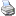# Arithmetic Operatorsbacklinks... CindyScript CSFundamentals String Operators Table of Contents Vectors and Matrices English 日本語

## Arithmetic Operators

The following section summarizes all functions and operators that can be applied to numbers. There are also many other mathematical operations, and these can be found in the sections Vectors and Matrices, Geometric Operators, and Function Plotting.

### Infix Operators

The elementary mathematical operators +, -, *, /, ^ are accessible in a straightforward manner. They can be applied to numbers and lists. Their particular meaning depends on the type of objects to which they are applied. For example, 5+7 evaluates to 12, while [2,3,4]+[3,-1,5] evaluates to [5,2,9]. Usually all these operators apply to real numbers as well as to complex numbers.

#### The addition operator: <expr>+<expr>

Description: Numbers (integers, real, complex) can be added with the + operator. Lists having the same structure can also be added; then the addition is carried out component wise.

 Code Result 7 + 8 15 2.3 + 5.9 8.2 [2,3,4] + [3,4,6] [5,7,10] [2,3,[1,2]] + [3,4,[1,3]] [5,7,[2,5]]

#### The subtraction operator: <expr>-<expr>

Description: Numbers (integers, real, complex) can be subtracted with the - operator. Lists of the same shape can also be subtracted. The subtraction is then performed componentwise. Furthermore, the - operator can be used as a unary minus.

 Code Result 7 - 8 -1 8.3 - 5.9 2.4 [2,6,4] - [3,4,6] [-1,2,-2] [5,3,[1,2]] - [3,4,[1,3]] [2,-1,[0,-1]]

#### The multiplication operator: <expr>*<expr>

Description: Numbers (integers, real, complex) can be multiplied with the * operator. Lists that represent numerical vectors or numerical matrices can also be multiplied if the dimensions admit a reasonable mathematical operation. See the examples for further description.

 Code Result 7 * 8 56 (integer multiplication) (1+i) * (2+i) 1+3*i (multiplication of complex numbers) 2 * [5,3,2] [10,6,4] (scalar multiplication of number and vector) [5,3,2] * 2 [10,6,4] (scalar multiplication of number and vector) [2,2,3] * [3,4,6] 32 (scalar product of two vectors) (x_1,x_2,…,x_n)*(y_1,y_2, …,y_n)=(x_1*y_1+…+x_n*y_n)) [[1,2],[3,4]] * [1,2] [5,11] (matrix times vector) [1,2] * [[1,2],[3,4]] [7,10] (vector times matrix) [[1,2],[3,4]] * [[1,2],[3,4]] [[7,10],[15,22]] (product of two matrices)

#### The division operator: <expr>/<number>

Description: Numbers (integers, real, complex) can be divided with the / operator.
Also, a vector can be divided by a number.

 Code Result 56 / 8 7 [6,8,4] / 2 [3,4,2]

#### The power operator: <expr>^<expr>

Description: A number (integer, real, complex) can be taken to the power of another number (integer, real, complex). Note that not only integer powers are allowed. In a^b the exponent b can
be an arbitrary real or complex number. Formally, the expression exp(b*ln(a)) is calculated.
Since ln(…) is defined only up to a period of 2*pi, the expression a^b is in general multivalued. For noninteger values of b only one principal value of a^b will be returned.

 Code Result 5^2 25 5^(-1) 0.2 2^(1/2) 1.4142…

#### The degree operator: <number>°

This operator multiplies any number by the constant pi/180 . This makes possible angle conversion from degrees to radians.

 Code Result 180° 3.1415… cos(180°) -1

#### The absolute value operator: |<number>|

Description: This operator calculates the absolute value of an object. The object may be a simple number, a complex number, or a vector.

It is not allowed to use the |...| operator in a nested way, since such expressions can be syntactically ambiguous.

 Code Result |-5| 5 |(3,4)| 5 |1+i| 1.4142…

#### The distance operator: |<number>,<number>|

Description: One can use |...| with two arguments, in which case this operator calculates the distance between the two objects. The objects may be simple numbers, complex numbers, or vectors. However, they must be of the same type.

It is not allowed to use the |...,...| operator in a nested way, since such expressions can be syntactically ambiguous.

 Code Result |-5,8| 13 |(1,1),(4,5)| 5

### Functional Operators

The following operators can be applied to numbers (integer, real complex).
Some of them can also be applied to vectors.

### Arithmetic Functions

#### Exponentiation: pow(<expr1>,<expr2>)

Description: These operators are binary functions equivalent to the operators like +, -, *, /, and ^.

 Code Result add(5,6) 11 pow(6,2) 36 mod(23,4) 3 add([1,2],[3,4]) (4,6) mult(2,[3,4]) (6,8) mult([4,5],[3,4]) 32

#### Modulo: mod(<expr1>,<expr2>)

Description: The mod function calculates the remainder of <expr1> if divided by <expr2>.

### Standard Functions

#### Natural logarithm: log(<expr>)

Description: These functions map numbers to numbers. Complex numbers are fully supported.

### Trigonometric Functions

The standard trigonometric functions are available through the following operators:

#### Angle of a vector: arctan2(<vec>)

The arc operators are in principle multivalued. However, the operator returns only one principal value, for which the real value is between +pi and -pi.

 Code Result sin(pi) 0 arccos(-1) 3.1415… arctan2(1,1) 45° arctan2(-1,-1) -135°

### Numeric Functions

#### Conjugate of a complex number: conjugate(<expr>)

Description: For complex numbers the operators round, floor, and ceil are applied to the real and imaginary parts separately.

The function abs calculates the norms of numbers, complex numbers, vectors, etc. All other functions can also be applied to lists, in which case they are applied component wise.

 Code Result round(4.3) 4 round([3.2,7.8,3.1+i*6.9]) [3,8,3+i*7] abs([1,3,1,2,1]) 4 floor(4.8) 4

### Random Number Operators

The following operators generate pseudo random numbers.

#### Uniformly distributed random integer number between 0 and <number>: randomint(<number>)

Description: The random generators also accept negative and complex numbers as arguments. For example, random(-5) generates a random number between -5 and 0; randomint(6+i*10) generates a random complex number for which the real part is an integer between 0 and 6 and the imaginary part is an integer between 0 and 10.

#### Initialize the random generator: seedrandom(<number>)

Description: The pseudo random generator will always produce unforeseeable new random numbers. If for some reason one wants the same random numbers to be generated for different runs of a script, one can use the seedrandom(<number>) operator. After this function is invoked with a certain integer, the same sequence of random numbers will be deterministically generated. Each seeding integer produces a different sequence of random numbers.